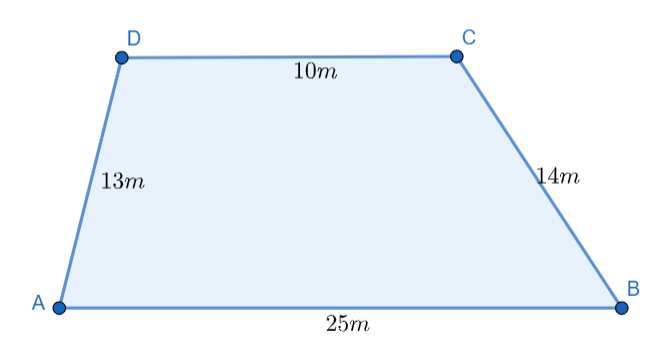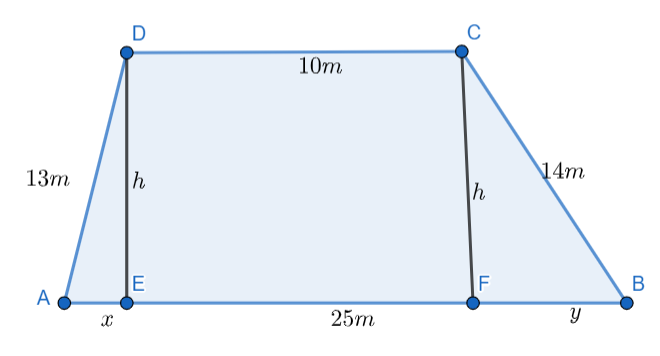Courses
Courses for Kids
Free study material
Free LIVE classes
MoreLIVE
Join Vedantu’s FREE Mastercalss

# A field is in the shape of a trapezium whose parallel sides are $25\,m$ and $10\,m$. The non-parallel sides are $14\,m$ and $13\,m$. Find the area of the field.Verified
359.4k+ views
Hint: The given question is related to the area of a trapezium. To find the distance between parallel sides, draw perpendiculars from the vertices to the opposite parallel side and use Pythagoras theorem in the triangles formed.

Consider the trapezium $ABCD$ with $AB\parallel CD$. The lengths of the parallel sides are given as $25\,m$ and $10\,m$. So, $AB=25\,m$ and $CD=10\,m$. The lengths of the non-parallel sides are given as $14\,m$ and $13\,m$. So, $AD=13\,m$ and $BC=14\,m$.We are asked to find the area of this trapezium. We know, if $a$ and $b$ are the lengths of the parallel sides and $h$ is the distance between the parallel sides of a trapezium, then its area is given as $Area=\dfrac{1}{2}\left( a+b \right)h$. In the given problem, we know the lengths of the parallel sides but we do not know the distance between the parallel sides.
Let’s draw two perpendiculars on the side $AB$, one from the vertex $D$ and the other from the vertex $C$. Let these perpendiculars be $DE$ and $CF$ respectively. We know the distance between the parallel sides is the length of the perpendicular line between them. So, $DE=CF=h$, where $h$ is the distance between the parallel sides of the trapezium.Let $AE=x\,m$ and $FB=y\,m$. $DE$ and $CF$ are two perpendiculars between two parallel lines. So, they must be parallel to each other. So, the distance between them should be constant. So, $EF=CD=10\,m$. So, $x+y+10=25$.
$\Rightarrow x+y=15.....(i)$
Now, in $\Delta AED$ , $DE\bot AE$. So, $\Delta AED$ is a right-angled triangle. Applying Pythagoras theorem, we get ${{x}^{^{2}}}+{{h}^{2}}={{13}^{2}}$.
$\Rightarrow {{x}^{2}}+{{h}^{2}}=169.....(ii)$
In $\Delta CBF$ , $CF\bot BF$. So, $\Delta CBF$ is a right-angled triangle. Applying the Pythagoras theorem, we get ${{y}^{2}}+{{h}^{2}}={{14}^{2}}$.
$\Rightarrow {{y}^{2}}+{{h}^{2}}=196.....(iii)$
Now, from equation $(i)$, we have $x+y=15$. $\Rightarrow x=15-y$
Substituting $x=15-y$ in equation $(ii)$, we get ${{\left( 15-y \right)}^{2}}+{{h}^{2}}=169$.
$\Rightarrow 225+{{y}^{2}}-30y+{{h}^{2}}=169$
$\Rightarrow {{y}^{2}}-30y+{{h}^{2}}=169-225$
$\Rightarrow {{y}^{2}}-30y+{{h}^{2}}=-56$
$\Rightarrow {{h}^{2}}=30y-{{y}^{2}}-56$
Substituting ${{h}^{2}}=30y-{{y}^{2}}-56$ in equation $(iii)$, we get ${{y}^{2}}+30y-{{y}^{2}}-56=196$
$\Rightarrow 30y=252$
$\Rightarrow y=8.4$
Now, substituting $y=8.4$ in ${{h}^{2}}=30y-{{y}^{2}}-56$, we get ${{h}^{2}}=30\left( 8.4 \right)-{{\left( 8.4 \right)}^{2}}-56$.
$\Rightarrow {{h}^{2}}=252-70.56-56$
$\Rightarrow {{h}^{2}}=125.44$
$\Rightarrow h=11.2$
So, the distance between the parallel sides is $11.2\,m$.
Now, we have the values of the lengths of parallel sides as well as the distance between the parallel sides.
So, the area of the trapezium is given as $Area=\dfrac{1}{2}\left( 10+25 \right)\times 11.2=196\,{{m}^{2}}$ .
So, the area of the field is $196\,{{m}^{2}}$.

Note: While making substitutions, make sure that the substitutions are done correctly and no sign mistakes are present. Sign mistakes can cause the final answer to be wrong.
Last updated date: 20th Sep 2023
Total views: 359.4k
Views today: 5.59k